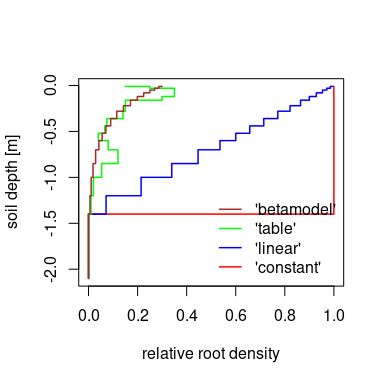# Model control options and parameters

## Introduction

This vignette demonstrates the use of different model control option setting (options_90-argument of runLWFB90()) and parameters (param_b90-argument).

Aside from the basic technical information of the simulation (startdate, enddate, fornetrad, prec_interval and correct_prec), the model control options control the annual course of leaf area index (lai_method), the phenology models to use (budburst_method, leaffall_method), the input and interpolations of annual stand properties (standprop_input, standprop_interp, use_growthperiod) and which root density depth distribution function to use (root_method). The interplay of options and parameters is shown briefly in the following paragraphs, by describing how options and parameters are passed from the options_b90 and param_b90 arguments to the individual functions that are called from within run_LWFB90().

For this purpose, we create basic lists of model control options and parameters, as well as soil and climate objects.

library(LWFBrook90R)
library(data.table)

options_b90 <- set_optionsLWFB90()
param_b90 <- set_paramLWFB90()

## Aboveground vegetation characteristics

### Intra-annual variation of leaf area index

The default parameter set (created with set_paramLWFB90()) represents a deciduous forest stand, without leafs in winter and maximum leaf area index in summer. The maximum leaf area index is defined by the parameter param_b90$maxlai, the minimum value in winter is internally calculated as a fraction (param_b90$winlaifrac) of param_b90$maxlai. The basic shape of the intra-annual leaf area index dynamics can be selected by the option options_b90$lai_method. The default setting 'b90' makes use of the parameters budburstdoy, leaffalldoy, emergedur and leaffalldur, that define the dates of budburst and leaffall, and the durations of leaf unfolding and leaf shedding until maxlai, and respectively winlaifrac in winter are reached. Within run_LWFB90(), the parameters are passed to make_seasLAI() that constructs the daily timeseries of leaf area index development for a single year:

LAI_b90 <-  make_seasLAI(method = options_b90$lai_method, year = 2003, maxlai = param_b90$maxlai,
winlaifrac = param_b90$winlaifrac, budburst_doy = param_b90$budburstdoy,
leaffall_doy = param_b90$leaffalldoy, emerge_dur = param_b90$emergedur,
leaffall_dur = param_b90$leaffalldur) make_seasLAI() also provides other shape functions that require additional parameters. For example, the option lai_method = 'linear' uses value pairs of day-of-year and leaf area index as fraction of maxlai passed from parameters param_b90$lai_doy and param_b90$lai_frac. The doy/value-pairs are then used to interpolate the intra-annual course of leaf area index to a daily time series. options_b90$lai_method <- "linear"
param_b90$lai_doy <- c(1,110,117,135,175,220,250,290,365) param_b90$lai_frac <- c(0.1,0.1,0.5,0.7,1.2,1.2,1.0,0.1,0.1)
LAI_linear <-  make_seasLAI(method = options_b90$lai_method, year = 2003, maxlai = param_b90$maxlai,
lai_doy = param_b90$lai_doy , lai_frac = param_b90$lai_frac)

A third shape-option for the intra-annual variation of leaf area index is called ‘Coupmodel’ and uses the interpolation method as implemented in the ‘Coupmodel’ (Jansson and Karlberg 2004). With lai_method ='Coupmodel, form parameters for leaf unfolding and leaf fall (shp_budburst, shp_leaffall), and the date when leaf area is at its maximum (shp_optdoy) are required, in addition to the parameters required by lai_method = 'b90'.

options_b90$lai_method <- "Coupmodel" param_b90$shp_budburst <- 0.5
param_b90$shp_leaffall <- 5 param_b90$shp_optdoy <- 180
LAI_coupmodel <-  make_seasLAI(method = options_b90$lai_method, year = 2003, maxlai = param_b90$maxlai,
budburst_doy = param_b90$budburstdoy, leaffall_doy = param_b90$leaffalldoy,
shp_budburst = param_b90$shp_budburst, shp_leaffall = param_b90$shp_leaffall,
shp_optdoy = param_b90$shp_optdoy) A plot of all three methods shows the roles of the different parameters: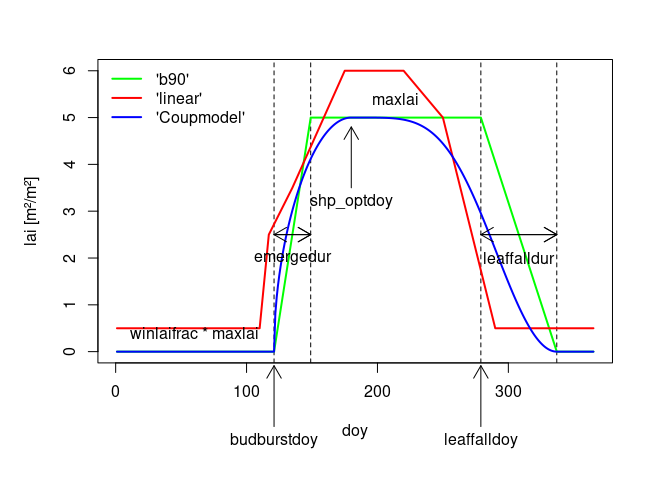Methods featured by make_seasLAI() ### Inter-annual variation of leaf area index By passing a single value via param_b90$maxlai we used the same maximum leaf area index for each year of the simulation period. In order to incorporate between-year variation of the leaf area index, we can simply assign vectors of values for each year of the simulation period to any of the parameters used by function make_seasLAI(). In the following example, we pass three values for maxlai and shp_optdoy, to get different seasonal courses of leaf area index for the three years of the simulation period. Additionally, we add variation to the dates of budburst, by assigning a vector of values to the parameter budburstdoy.

years <- 2001:2003
param_b90$maxlai <- c(4,6,5) param_b90$shp_optdoy <- c(210,180,240)
param_b90$shp_budburst <- c(3,1,0.3) param_b90$budburstdoy <- c(100,135,121)
lai_variation <- make_seasLAI(method = options_b90$lai_method, year = years, maxlai = param_b90$maxlai,
budburst_doy = param_b90$budburstdoy, leaffall_doy = param_b90$leaffalldoy,
shp_budburst = param_b90$shp_budburst, shp_leaffall = param_b90$shp_leaffall,
shp_optdoy = param_b90$shp_optdoy)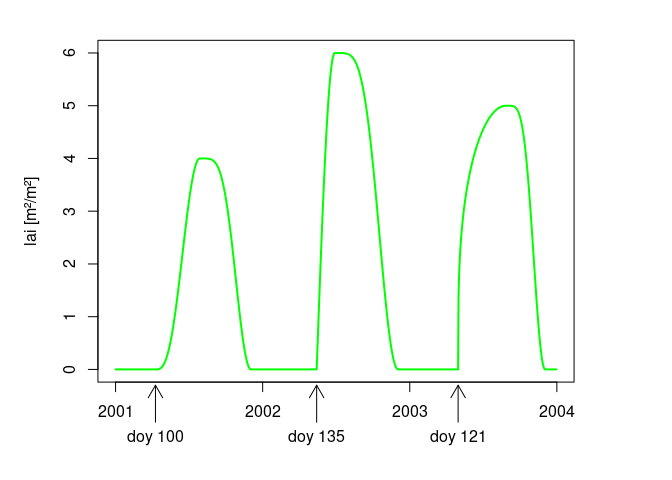Options and parameters affecting interannual variation of leaf area index. Beside the obvious between-year variation of maximum leaf area index, we can also see the effect of the shape parameter for the leaf unfolding phase shp_budburst. Values greater 1 result in concave, values below 1 in convex functions, while values of 1 give linear progressions. The budburst day-of-year is varying as specified in the parameters, but can also be estimated using temperature based phenology models. By selecting other settings than the default options_b90$budburst_method = 'fixed' and options_b90$leaffall_method = 'fixed', the vegperiod() function of the ‘vegperiod’-Package is called from within run_LWFB90. budburstdoy and/or leaffalldoy are then calculated for each year from the climate data using the desired methods. See vegperiod for a list of available models. The estimated values for budburstdoy and/or leaffalldoy can be found in the param_b90 list element of the results object after the simulation. ### Other plant properties (height, sai, densef) Like the leaf area index parameters and budburst/leaffall-dates, it is also possible to provide vectors of values for stand height (height), stem area index (sai), and stand density (densef) to generate between-year variation of stand characteristics. From the yearly values, daily values are interpolated using the function approx_standprop(). The approx.method- argument of the function defines how to interpolate the yearly values passed by y. Within run_LWFB90(), the option options_b90$standprop_interp is passed to the approx.method- argument of approx_standprop. The default interpolation method standprop_interp = 'constant' results in a yearly changing step function, while standprop_interp = 'linear' interpolates the values:

# constant 'interpolation'
options_b90$standprop_interp <- 'constant' param_b90$height <- c(20.2,20.8,21.3)
simyears <- 2002:2003
height_c <- approx_standprop(x_yrs=years,
y = param_b90$height, approx.method = options_b90$standprop_interp)

# linear interpolation
options_b90$standprop_interp <- 'linear' param_b90$height_ini <- 19.1
height_l <- approx_standprop(x_yrs=years,
y = param_b90$height, y_ini = param_b90$height_ini,
approx.method = options_b90$standprop_interp) For linear interpolation, additional parameters height_ini, sai_ini, densef_ini have to be provided to run_LWFB90() via the param_b90-argument. These parameters define the values at the beginning of the simulation, to which the value of the first year is interpolated to. By default, the yearly values are interpreted to be valid at December 31st of the respective years, so that the interpolated timeseries are linearly increasing or decreasing during the whole year. In order to constrain the interpolation to the growth period only, the option options_b90$use_growthperiod was introduced, which requires the arguments startdoy and enddoy, when set to TRUE. Then, values decrease or increase between budburst and leaffall only, and remain constant during winter.

options_b90$use_growthperiod <- TRUE height_l_gp <- approx_standprop(x_yrs = years, y = param_b90$height,
y_ini = param_b90$height_ini, use_growthperiod = options_b90$use_growthperiod,
startdoy = param_b90$budburstdoy, enddoy = param_b90$leaffalldoy,
approx.method = options_b90$standprop_interp) The following plot explains the differences between the interpolated timeseries of stand height using the different options and parameters: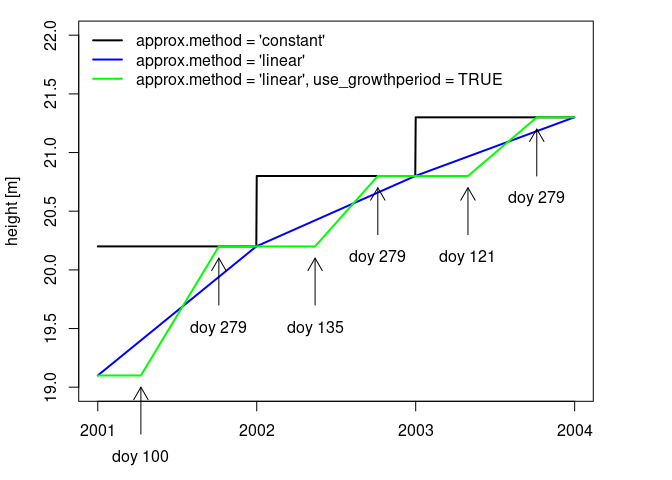Interpolated stand height derived from parameters using approx_standprop() Another option for incorporating between-year variation of plant properties is to provide a data.frame with yearly values of height, maxlai, sai, densef and age as list item standprop_table in param_b90. To take effect, the option options_b90$standprop_input has to be set to 'table'. In this case, the values passed via parameters height, sai, densef and age_ini are ignored. As maxlai is also provided via the table, the maxlai value from parameters is ignored as well, while the other parameters that affect intra-annual leaf area development (e.g., shp_budburst) are still active.

For demonstration purposes we use the table slb1_standprop, that contains observed stand data of the Solling Beech Experimental site from 1966 to 2014, along with estimated leaf and stem area index derived using allometric functions. For creating the daily timeseries of stand properties, we use run_LWFB90(), and make use of the option to not run the model (run = FALSE), but only return the model input.

#Extend simulation period
options_b90$startdate <- as.Date("1980-01-01") options_b90$enddate <- as.Date("1999-12-31")

soil <- cbind(slb1_soil, hydpar_wessolek_tab(texture = slb1_soil$texture)) #set up options for table input options_b90$standprop_input <- 'table'
param_b90$standprop_table <- slb1_standprop # Set up dynamic budburst and leaf fall options_b90$budburst_method <- "Menzel"
options_b90$leaffall_method <- "vonWilpert" param_b90$budburst_species <- "Fagus sylvatica"

#run LWF-Brook90 without simulation
standprop_daily <- run_LWFB90(options_b90 = options_b90,
param_b90 = param_b90,
climate = slb1_meteo,
soil = soil,
output = output,
run = FALSE)$standprop_daily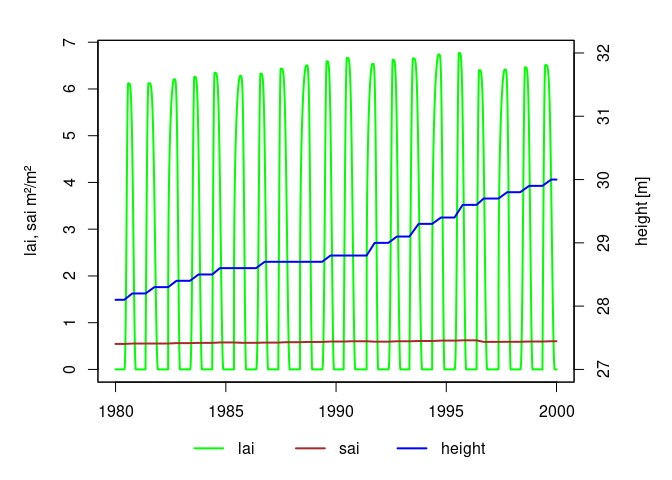Stand properties generated using table input of annual stand characteristics ## Root density depth distribution The root depth density depth distribution can either be provided in the column rootden of the soil- argument of run_LWFB90(), or can be derived from parameters using the function make_rootden(). In order to use root density as specified in the soil data, the root_method element of the options_b90-list has to be set to 'soilvar'. Other method names are passed to make_rootden(). Currently, the function provides four methods to assign values of relative root density to a vector of soil depths. The default method 'betamodel' uses the model of Gale & Grigal (-Gale and Grigal (1987)), which is of the form $$y = 1- \beta^d$$, where $$y$$ is the cumulative root fraction down to soil depth $$d$$ and $$\beta$$ is the depth coefficient. Larger values of $$\beta$$ correspond to a greater proportion of roots in deeper soil layers: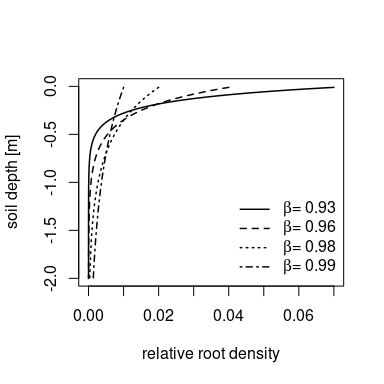For larger values of $$\beta$$, the root density will reach zero only in very deep soil layers. In order to set the root density to zero at any desired soil depth, the parameter maxrootdepth was defined. With this parameter, the root density is set to zero in all soil layers that lie deeper than maxrootdepth. Within run_LWFB90(), the function is called in the following way: param_b90$maxrootdepth <- -1.4
options_b90$root_method <- "betamodel" roots_beta <- make_rootden(soilnodes = c(max(slb1_soil$upper), slb1_soil$lower), maxrootdepth = param_b90$maxrootdepth,
beta = param_b90$betaroot, method = options_b90$root_method)

A second option to define the root distribution for the soil layers is to provide value pairs of soil depth and root density in a data.frame and assign it to the rootden_table-entry in param_b90. As an example, we set up a hypothetical root density depth distribution:

options_b90$root_method <- 'table' param_b90$rootden_table <- data.frame(
upper = c(0.03,0,-0.02, -0.15, -0.35, -0.5, -0.65,-0.9,-1.1,-1.3),
lower = c(0,-0.02, -0.15, -0.35, -0.5, -0.65,-0.9,-1.1,-1.3,-1.6),
rootden = c(10,15, 35, 15, 7.5, 4, 12, 2, 2, 0))
roots_table <- make_rootden(soilnodes = c(max(slb1_soil$upper), slb1_soil$lower),
method = options_b90$root_method, rootdat = param_b90$rootden_table)

A third option generates a linear root density depth distribution, with the maximum at the uppermost soil layer and a root density of 0 at maxrootdepth. If the parameter relrootden is provided, the first element of the vector is used as the maximum, otherwise the interpolation is made between 0 and 1. The last option returns a uniform root distribution, with the first vector-element of relrootden (if provided) as value for all layers down to maxrootdepth.

options_b90$root_method <- 'linear' roots_linear <- make_rootden(soilnodes = c(max(slb1_soil$upper), slb1_soil$lower), maxrootdepth = param_b90$maxrootdepth,
method = options_b90$root_method) options_b90$root_method <- 'const'
roots_constant <- make_rootden(soilnodes = c(max(slb1_soil$upper), slb1_soil$lower),
maxrootdepth = param_b90$maxrootdepth, method = options_b90$root_method)